RRB JE CBT 1 - Full Test 1

# RRB JE CBT 1 - Full Test 1

Test Description

## 100 Questions MCQ Test RRB JE (Railways Junior Engineer) CBT Mock Test Series | RRB JE CBT 1 - Full Test 1

RRB JE CBT 1 - Full Test 1 for Railways 2023 is part of RRB JE (Railways Junior Engineer) CBT Mock Test Series preparation. The RRB JE CBT 1 - Full Test 1 questions and answers have been prepared according to the Railways exam syllabus.The RRB JE CBT 1 - Full Test 1 MCQs are made for Railways 2023 Exam. Find important definitions, questions, notes, meanings, examples, exercises, MCQs and online tests for RRB JE CBT 1 - Full Test 1 below.
Solutions of RRB JE CBT 1 - Full Test 1 questions in English are available as part of our RRB JE (Railways Junior Engineer) CBT Mock Test Series for Railways & RRB JE CBT 1 - Full Test 1 solutions in Hindi for RRB JE (Railways Junior Engineer) CBT Mock Test Series course. Download more important topics, notes, lectures and mock test series for Railways Exam by signing up for free. Attempt RRB JE CBT 1 - Full Test 1 | 100 questions in 90 minutes | Mock test for Railways preparation | Free important questions MCQ to study RRB JE (Railways Junior Engineer) CBT Mock Test Series for Railways Exam | Download free PDF with solutions
 1 Crore+ students have signed up on EduRev. Have you?
RRB JE CBT 1 - Full Test 1 - Question 1

### Khan Abdul Ghaffar was also known by which name?

Detailed Solution for RRB JE CBT 1 - Full Test 1 - Question 1
•  Khan Abdul Ghaffar Khan was also known as Badshah Khan was a non-violent activist and close confidante of Mahatma Gandhi.
•  He was also known as Frontier Gandhi.
• He led his non-violent Khudai Khidmatgar movement against the British Raj in the 1930s and 1940s.
RRB JE CBT 1 - Full Test 1 - Question 2

### Which of the following is related to the Tinkathia Pratha?

Detailed Solution for RRB JE CBT 1 - Full Test 1 - Question 2
• Tinkathia System or Tinkathia Pratha was a system under which the native peasants of Champaran were forced by the European planters to use 3/20th of their land holding to cultivate Indigo.
• The European planters practiced illegal methods to grow indigo, however, with the help of Rajendra Prasad and Gandhiji the peasants of Champaran organised Satyagraha and abolished the system.
RRB JE CBT 1 - Full Test 1 - Question 3

### The annulment of Partition of Bengal was done by ________.

Detailed Solution for RRB JE CBT 1 - Full Test 1 - Question 3

Lord Hardinge, the Viceroy of India from 1910 to 1916 ,had annulled the partition of Bengal in 1911.

Note: The partition of Bengal was done by Lord Curzon on 16th October 1905 and The annulment of Partition of Bengal was done by Lord Hardinge in 1911.

RRB JE CBT 1 - Full Test 1 - Question 4

Which of the following newspapers is NOT related to ‘Madan Mohan Malaviya’?

Detailed Solution for RRB JE CBT 1 - Full Test 1 - Question 4
• Comrade is not related to Madan Mohan Malaviya who had been served as the president of Indian National Congress for four times.
• Comrade was published and edited by Maulana Mohammad Ali between 1911 and 1914.
RRB JE CBT 1 - Full Test 1 - Question 5

Singar Chouri peak on the northern slopes of the Vindhyan escarpment situated near Indore is the source of which river?

Detailed Solution for RRB JE CBT 1 - Full Test 1 - Question 5

The 960 kilometres (600 mi) long Chambal River originates from the Singar Chouri peak on the northern slopes of the Vindhyan escarpment, 15 kilometres (9.3 mi) West-South-West of Mhow in Indore District, Madhya Pradesh state.

• Chambal River is a tributary of Yamuna River.
• Right Bank Tributaries - Parbati, Kali Sindh, Shipra.
• Left Bank Tributaries- Gandhi Sagar, Rana Pratap Sagar, Jawahar Sagar, Kota Barrage.
RRB JE CBT 1 - Full Test 1 - Question 6

Which state is the largest producer of Mica in India?

Detailed Solution for RRB JE CBT 1 - Full Test 1 - Question 6

Andhra Pradesh is the largest producer of mica in India followed by Rajasthan and Jharkhand. Mica is used in paints.

RRB JE CBT 1 - Full Test 1 - Question 7

Which among the following states has the largest railway network in India?

Detailed Solution for RRB JE CBT 1 - Full Test 1 - Question 7
• Uttar Pradesh has the largest railway network in the country.
• However, it has the sixth-highest railway density among the states in India.
• The state has the railway network of 9077.45 km connecting it with other parts of the country.
RRB JE CBT 1 - Full Test 1 - Question 8

Hindu Pilgrimage center Sabarimala is located in which state?

Detailed Solution for RRB JE CBT 1 - Full Test 1 - Question 8
• Hindu Pilgrimage center Sabarimala is located at the Periyar Tiger Reserve in the Western Ghat mountain ranges of Pathanamthitta district, Perunad, Kerala.
• It is one of the world’s largest annual pilgrimages with over 40-50 million devotees visiting each year.
RRB JE CBT 1 - Full Test 1 - Question 9

Which layer of the atmosphere has the maximum concentration of Ozone gas?

Detailed Solution for RRB JE CBT 1 - Full Test 1 - Question 9
• Stratosphere layer of atmosphere has the maximum concentration of Ozone gas.
• Approximately 10 per cent of Ozone layer is present in Troposphere and rest of the 90 per cent found in Stratosphere layer of atmosphere..
• Ozone layer in Stratosphere atmosphere absorbs most of the ultraviolet radiation from the Sun.
• Stratosphere is the second layer of atmosphere whereas Troposphere is the lowest layer of the atmosphere.
• The Thermosphere is the layer of the Earth's atmosphere is above the Mesosphere.
• There are five layers of atmosphere namely- Troposphere, Stratosphere, Mesosphere, Thermosphere and Exosphere.
RRB JE CBT 1 - Full Test 1 - Question 10

The greenhouse gases, otherwise called radioactively active gases include

Detailed Solution for RRB JE CBT 1 - Full Test 1 - Question 10
• The gases which contribute to the greenhouse effect by absorbing infrared radiations are known as the greenhouse gases.
• The greenhouse gases are also termed as the radioactively active gases which include Carbon Dioxide (CO2), Methane (CH4), Nitrous Oxide (N2O), etc.
RRB JE CBT 1 - Full Test 1 - Question 11

Who among the following is the current Chief Minister of Manipur?

Detailed Solution for RRB JE CBT 1 - Full Test 1 - Question 11

N. Biren Singh is the incumbent Chief Minister of Manipur. He is a member of BJP. He won the 2017 Manipur Legislative Assembly Election from Heingang Assembly Constituency.

RRB JE CBT 1 - Full Test 1 - Question 12

Ganga Gram Yojana was launched by which of the following ministries?

Detailed Solution for RRB JE CBT 1 - Full Test 1 - Question 12
• The Ganga Gram Yojana was launched in January 2016 at Village Puth in Hapur district of Uttar Pradesh by the Ministry of Drinking Water and Sanitation.
• This scheme aims to develop 1600 villages of UP which are situated along the banks of river Ganga.
RRB JE CBT 1 - Full Test 1 - Question 13

Who is the author of "Brief Answers to the Big Questions"?

Detailed Solution for RRB JE CBT 1 - Full Test 1 - Question 13
• "Brief Answers to the Big Questions" is a popular-science book written by Stephen Hawking.
• The book promotes the view that science is very important in helping to solve the problems on Earth.
RRB JE CBT 1 - Full Test 1 - Question 14

Who has won the FIFA Golden Ball for the best player at the World Cup 2018?

Detailed Solution for RRB JE CBT 1 - Full Test 1 - Question 14

Croatia captain Luka Modric has won the FIFA Golden Ball for the best player at the World Cup 2018. England national football captain Harry Kane was awarded the Golden Boot for scoring most goals (6) in FIFA World Cup 2018. Belgium’s Thibaut Courtois won the Golden Glove as the best goalkeeper of FIFA World Cup 2018.

RRB JE CBT 1 - Full Test 1 - Question 15

The 'Malabar' is a naval exercise between which among the following countries?

Detailed Solution for RRB JE CBT 1 - Full Test 1 - Question 15
• Malabar Exercise was started as Indo-US bilateral naval war drill in 1992.
• Japan had formally joined the exercise as permanent participant in 2015, making it trilateral exercise.
• The primary aim of this multilateral exercise is to increase interoperability amongst navies of three countries and develop common understanding of procedures for Maritime Security Operations and deepen defence relations between them.
RRB JE CBT 1 - Full Test 1 - Question 16

Which of the following lens always forms a virtual, erect and diminished image?

Detailed Solution for RRB JE CBT 1 - Full Test 1 - Question 16
• A concave lens is a lens that possesses at least one surface that curves inwards.
• It is a diverging lens, meaning that it spreads out light rays that have been refracted through it.
•  A concave lens is thinner at its centre than at its edges and produces an erect and diminishing image.
RRB JE CBT 1 - Full Test 1 - Question 17

The effect of a force on the body is measured only in terms of its ________.

Detailed Solution for RRB JE CBT 1 - Full Test 1 - Question 17
• Impulse is defined as the integral of force over the time interval for which it acts.
• If a moving object is stopped by an impact, the change in momentum of the object occurs. This change in momentum is called the impulse.
• Impulse is a vector quantity and the impulse force on the body is measured in terms of its impulse force.
RRB JE CBT 1 - Full Test 1 - Question 18

If the momentum of a body is doubled, the kinetic energy is _____.

Detailed Solution for RRB JE CBT 1 - Full Test 1 - Question 18

The momentum of a body, P = mv, where m is the mass of the particle, and v is the velocity of the particle.

Momentum is directly proportional to velocity.

The kinetic energy of a particle is given by the equation, KE = (1/2)mv2.

KE = (1/2)mv2

When the momentum of a body is doubled, then the velocity will be doubled.

⇒ KE = (1/2) × m × (2v)2 = 4 × (1/2)mv2

Hence, If the momentum of a body is doubled, the kinetic energy becomes 4 times.

RRB JE CBT 1 - Full Test 1 - Question 19

A car of mass 300 kg is travelling along a horizontal road with a speed of 10m/s. The acceleration of car is 4/3 m/s2 . A resistive force of 100 N is opposing its motion. Find the power generated by the engine of the car in this case.

Detailed Solution for RRB JE CBT 1 - Full Test 1 - Question 19

To find the Power in this case, we must apply the following formula:

P= Fnet . v

We first need to find out the net force applied on the car.

Fnet = mass × acceleration + resistive force

= 300 × 4/3 + 100

= 400 + 100 = 500 N

Now, P= 500 × 10 = 5000 W = 5 kW

RRB JE CBT 1 - Full Test 1 - Question 20

Which of following property of a proton cannot change while it moves freely in a magnetic field?

Detailed Solution for RRB JE CBT 1 - Full Test 1 - Question 20
• The magnetic force acts perpendicular to the direction of motion of the proton, thus it does not change the speed but only its direction of motion
• This in turn means the velocity and momentum of the particle is changed.
RRB JE CBT 1 - Full Test 1 - Question 21

An object is said to be in what kind of motion if its speed keeps changing while moving along a straight line?

Detailed Solution for RRB JE CBT 1 - Full Test 1 - Question 21

A body is said to be in uniform motion if it travels equal distances in equal intervals of time. A body is said to have non-uniform motion if it travels unequal distances in equal intervals of time. These concepts are based on Newton’s three laws of motion.

RRB JE CBT 1 - Full Test 1 - Question 22

Within an environment, what is horizontal heat transfer called?

Detailed Solution for RRB JE CBT 1 - Full Test 1 - Question 22
• Conduction is the transfer of heat energy by direct contact.
• Convection is the movement of heat by actual motion of matter.
• Absorption is a physical or chemical phenomenon or a process in which atoms, molecules or ions enter some bulk phase, liquid or solid material.
• Advection is defined as the transfer of heat or matter by the flow of a fluid, especially horizontally in the atmosphere.

Hence, 4 is the correct option.

RRB JE CBT 1 - Full Test 1 - Question 23

Pick out the scalar quantity from the followings.

Detailed Solution for RRB JE CBT 1 - Full Test 1 - Question 23

A scalar quantity is a one-dimensional quantity consisting of only magnitude and devoid of direction.

• Work is an example of scalar quantity as it has no precise direction attached to it making it a one-dimensional quantity.
• Other examples of scalar quantities include time, volume, speed, mass, temperature, distance, entropy, energy etc.
RRB JE CBT 1 - Full Test 1 - Question 24

What is meant by residual volume of lungs?

Detailed Solution for RRB JE CBT 1 - Full Test 1 - Question 24

The residual volume is volume of air left inside the lungs after exhalation, this is the minimum volume of the lungs that remains even after air is pushed out of the lungs.

RRB JE CBT 1 - Full Test 1 - Question 25

Where are the mucous glands located in the respiratory system?

Detailed Solution for RRB JE CBT 1 - Full Test 1 - Question 25

The mucus glands are located in the lining of the nasal cavity. They secrete mucus. Mucus is a fluid that keeps the lining of the respiratory system moist and traps dust and other foreign bodies in it. It is removed by small hair structures called cilia towards the mouth.

RRB JE CBT 1 - Full Test 1 - Question 26

K-39 test is done to detect which of the following diseases?

Detailed Solution for RRB JE CBT 1 - Full Test 1 - Question 26

Kala Azar, also known as Visceral Leishmaniasis is a disease caused by protozoan parasites of the Leishmania genus. It is detected by the K-39 test. Typhoid is detected by the Widal test. ELISA test is done to detect AIDS.

RRB JE CBT 1 - Full Test 1 - Question 27

Which of the following is the highest source of protein?

Detailed Solution for RRB JE CBT 1 - Full Test 1 - Question 27

Soyabean has the highest source of protein content of 36 g, Grams contains 19 g protein content, sunflower protein content is 21 g and wheat‘s is 13.2 g.

RRB JE CBT 1 - Full Test 1 - Question 28

Night Blindness is caused by a deficiency of which of the following Vitamin?

Detailed Solution for RRB JE CBT 1 - Full Test 1 - Question 28

Night Blindness results in loss of vision in the dark and is caused by the deficiency of Vitamin A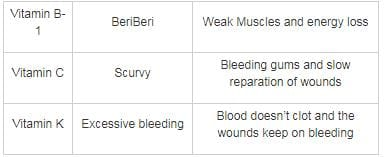RRB JE CBT 1 - Full Test 1 - Question 29

________ are pollution indicator plants

Detailed Solution for RRB JE CBT 1 - Full Test 1 - Question 29

Lichens are best indicator of pollution because lichens are very sensitive to sulphur dioxide pollution in the air. If air is very badly polluted with sulphur dioxide there may be no lichens present, just green algae may be found. If the air is clean, shrubby, hairy and leafy lichens become abundant. A few lichen species can tolerate quite high levels of pollution and are commonly found on pavements, walls and tree bark in urban areas. Lichens have also shown sensitivity to some other pollutants, such as heavy metals and other toxic gases in air.

RRB JE CBT 1 - Full Test 1 - Question 30

The other name for phloem is ________.

Detailed Solution for RRB JE CBT 1 - Full Test 1 - Question 30

Bast fibre (also called phloem fibre or skin fibre) is plant fibre collected from the phloem (the "inner bark", sometimes called "skin"). In vascular plants, phloem is the living tissue that transports the soluble organic compounds made during photosynthesis in particular sugar sucrose, to parts of the plant where needed.

RRB JE CBT 1 - Full Test 1 - Question 31

Where do most of the digestive process take place?

Detailed Solution for RRB JE CBT 1 - Full Test 1 - Question 31

The small intestine is the part of the intestines where 90% of the digestion and absorption of the food occurs, the other 10% taking place in the stomach and large intestine.

RRB JE CBT 1 - Full Test 1 - Question 32

Which one of the following is a widely used as solid lubricant?

Detailed Solution for RRB JE CBT 1 - Full Test 1 - Question 32
• Graphite is widely used as a solid lubricant.
• Graphite is crystalline, low density, and soft allotrope of carbon.
• Graphite is composed of loosely bound sheets of carbon atoms, giving it lubricating texture which makes it an effective lubricating agent.
RRB JE CBT 1 - Full Test 1 - Question 33

Which variety of glass is used for the manufacture of optical lenses?

Detailed Solution for RRB JE CBT 1 - Full Test 1 - Question 33

Optical glass, also known as flint glass, is softer than any other glass. It is clear and transparent. The main use of flint glass is in the manufacture of lenses, prisms and other optical instruments.

RRB JE CBT 1 - Full Test 1 - Question 34

Silicon possess how many valence electrons?

Detailed Solution for RRB JE CBT 1 - Full Test 1 - Question 34

Silicon possess 4 valence electron (2, 8, 4). It has 2 electron in first shell,eight electrons in the second shell, and four electrons in the third shell.Since the electrons in the third shell are the outermost electrons, silicon has four valence electrons.​

Its electronic configuration is written as 1s22s22p63s23p2.

RRB JE CBT 1 - Full Test 1 - Question 35

Heating of ore in the absence of air below its melting point is called?

Detailed Solution for RRB JE CBT 1 - Full Test 1 - Question 35
• Calcination is a thermal treatment process in the absence or limited supply of air or oxygen. This process is applied to ores and other solid materials to bring about a thermal decomposition.
• Reduction: Reduction is a chemical reaction that involves the gaining of electrons by one of the atoms involved in the reaction
• Oxidation: Oxidation is the loss of electrons during a reaction
• Catalyst: Catalyst is a substance added to change the rate of reaction but it does not take part in the final products
RRB JE CBT 1 - Full Test 1 - Question 36

Butane and isobutane are ______

Detailed Solution for RRB JE CBT 1 - Full Test 1 - Question 36

Isomers are molecules that have the same molecular formula but different chemical structures. Therefore Butane and isobutane would be called as isomers.

RRB JE CBT 1 - Full Test 1 - Question 37

Which of the following gases is used for artiﬁcial ripening of green fruits?

Detailed Solution for RRB JE CBT 1 - Full Test 1 - Question 37

Acetylene gas is used for artiﬁcial ripening of green fruits.

• It is produced when artificial ripening agent calcium carbide comes in contact with moisture.
• It has carcinogenic properties. Its compound formula is C₂H2.
• It is also used in the steel plants for the purpose of welding and cutting.
RRB JE CBT 1 - Full Test 1 - Question 38

Rock Salt contains which mineral?

Detailed Solution for RRB JE CBT 1 - Full Test 1 - Question 38
• Halite commonly known as rock salt, is a type of salt, the mineral (natural) form of sodium chloride (NaCl).
• Halite forms isometric crystals.
RRB JE CBT 1 - Full Test 1 - Question 39

Which among the following is added to commercial nitric acid to make it colored?

Detailed Solution for RRB JE CBT 1 - Full Test 1 - Question 39

Commercial nitric acid has a brown color due to dissolved NO(Nitrogen Dioxide), however pure nitric acid is colourless.

RRB JE CBT 1 - Full Test 1 - Question 40

Which country imposed the world's toughest law against plastic bags recently?

Detailed Solution for RRB JE CBT 1 - Full Test 1 - Question 40

Kenya imposed the world's toughest law against plastic bags recently.

• If they sell or use any plastic bags it would become a risk of imprisonment of up to four years or fines of \$40,000.
• Plastic is banned in more than 40 other countries including China, France, Rwanda, and Italy.
RRB JE CBT 1 - Full Test 1 - Question 41

Which is/are NOT Abiotic components?

I. Plants
II. Animals
III. Water

Detailed Solution for RRB JE CBT 1 - Full Test 1 - Question 41
• An abiotic component is a non-living component in the environment.
•  These components have a major influence on a living organism.
•  Abiotic components are water, sunlight, temperature, fire, heat, sunlight, oxygen, climate.
•   Water is an only abiotic component from the above-mentioned components.
RRB JE CBT 1 - Full Test 1 - Question 42

When was the Montreal protocol assigned to reduce the production of Chlorofluorocarbons?

Detailed Solution for RRB JE CBT 1 - Full Test 1 - Question 42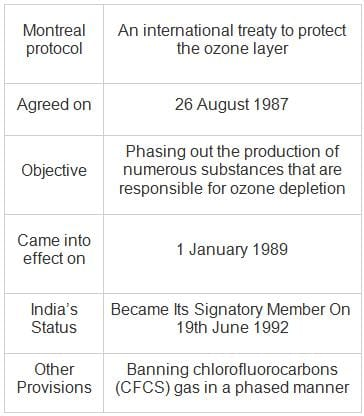RRB JE CBT 1 - Full Test 1 - Question 43

Due to large quantities of nutrients in water, an excessive growth of planktonic  ________ occurs.

Detailed Solution for RRB JE CBT 1 - Full Test 1 - Question 43
• Due to large quantities of nutrients in the water, excessive growth of planktonic algae occurs.
• Eutrophication is when a body of water becomes overly enriched with minerals and nutrients that induce excessive growth of plants and algae.
• However, it reduces the amount of oxygen in any water body since these organisms consume a lot of oxygen.
RRB JE CBT 1 - Full Test 1 - Question 44

The term ecosystem was first used by ________.

Detailed Solution for RRB JE CBT 1 - Full Test 1 - Question 44
• British ecologist A.G. Tansley used the term ecosystem for the first time in 1935.
• According to him, an ecosystem is a special category of physical system, which consists of organic as well as inorganic components in a stable manner, of various sizes and kinds.
RRB JE CBT 1 - Full Test 1 - Question 45

Which of the following countries has built an 'artificial sun'?

Detailed Solution for RRB JE CBT 1 - Full Test 1 - Question 45
• China has built an 'artificial sun' that reaches temperature six times that of the core of the sun.
• The reactor named 'Experimental Advanced Superconducting Tokamak' (EAST) is designed to replicate the processes of the sun as part of a project to turn hydrogen into cost-effective green energy.
• It involves fusion of hydrogen atoms together to form heavier elements, such as helium.
RRB JE CBT 1 - Full Test 1 - Question 46

In the following question, find the odd word from the given alternatives.

Detailed Solution for RRB JE CBT 1 - Full Test 1 - Question 46

Here Pisces, Gemini and Taurus are all Zodiac signs, while Arial is a name of font in MS office.

Thus, Arial is the odd word.

RRB JE CBT 1 - Full Test 1 - Question 47

In the following question, find the odd number pair from the given alternatives.

Detailed Solution for RRB JE CBT 1 - Full Test 1 - Question 47

Let’s check each option,

1) 18 × 8 = 144

2) 16 × 8 = 128

3) 23 × 8 = 184

4) 15 × 7 = 105

Thus the odd number pair is (15, 105).

RRB JE CBT 1 - Full Test 1 - Question 48

In the following question, find the related letters from the given alternatives.
SPAIN : USENT :: ? : LDTFT

Detailed Solution for RRB JE CBT 1 - Full Test 1 - Question 48

The logic here is as follows,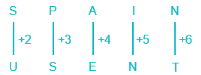Similarly,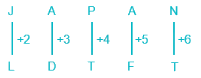Thus JAPAN is related to LDTFT.

RRB JE CBT 1 - Full Test 1 - Question 49

In the following question, find the related words from the given alternatives.
MRF : Madras Rubber Factory :: BMW : ?

Detailed Solution for RRB JE CBT 1 - Full Test 1 - Question 49

MRF is the acronym for ‘Madras Rubber Factory’, similarly BMW is the acronym for ‘Bavarian Motor Works'.

Thus, BMW is related to Bavarian Motor Works.

RRB JE CBT 1 - Full Test 1 - Question 50

In the following question, find the related number from the given alternatives.
27 : 198 :: 13 : ?

Detailed Solution for RRB JE CBT 1 - Full Test 1 - Question 50

The logic here is as follows,

27 × 7 = 189; 189 + 9 (i.e., 2 + 7) = 198

Similarly,

13 × 7 = 91; 91 + 4 (i.e., 1 + 3) = 95

Thus, 13 is related to 95.

RRB JE CBT 1 - Full Test 1 - Question 51

Find the wrong term in the following series.
2259, 2428, 2624, 2850, 3105, 3394

Detailed Solution for RRB JE CBT 1 - Full Test 1 - Question 51

2259 + 169 → 2259 + 132 = 2428;

2428 + 196 → 2428 + 142 = 2624;

2624 + 225 → 2624 + 152 = 2849;

2849 + 256 → 2849 + 162 = 3105;

3105 + 289 → 3105 + 172 = 3394

Hence, “2850” is the wrong term in the series, it should be 2849 in place of 2850.

RRB JE CBT 1 - Full Test 1 - Question 52

A series is given with one term missing. Select the correct alternative from the given ones that will complete the series
Elegant, Peanut, Scent, Tire, Number, ?

Detailed Solution for RRB JE CBT 1 - Full Test 1 - Question 52

The logic followed here is as follows,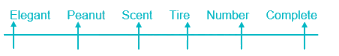The position of letter E in each term is moving forward by one place.

Thus, the next word in the series will be ‘Complete’.

RRB JE CBT 1 - Full Test 1 - Question 53

If in a certain code language, “SARA” is written as “NGKI”, then what will be the code for “DEBT”.

Detailed Solution for RRB JE CBT 1 - Full Test 1 - Question 53

The logic here is as follows,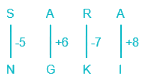Similarly,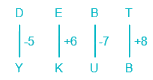Thus, DEBT is coded as YKUB.

RRB JE CBT 1 - Full Test 1 - Question 54

Study the following information carefully and answer the given question.

In a certain language,
“Subject of opinion” can be written as “7#C 2@O 7#O”
“Big Priority” can be written as “8@T 3#I”
“Impact is important” can be written as “2@I 9#N 6@C”
Then how is “Financial stability” can be written as ?

Detailed Solution for RRB JE CBT 1 - Full Test 1 - Question 54

Logic followed here is,

@ → even number of letter in a word

# → odd number of letter in a word

The number in each code is referred to as the number of letter in a word.

The last letter in each code is referred to as the second last letter of each word.

Financial can be written as → 9#A

Stability can be written as → 9#T

Hence, “9#T 9#A” is the correct answer.

RRB JE CBT 1 - Full Test 1 - Question 55

Study the following information carefully and answer the given questions.

There are 11 players playing a football match where 2 players got a red card. A is the goalkeeper standing at the eastern point of the ground. A is having the ball and he passes the ball to J who is in the north of A. Then J passes the ball to F who is to his north-west direction. F passes the ball to D who is to his south-west direction. D who is in the west direction of J passes the ball to J. Then J kick the ball so that it can reach to player E who is in the west of J. Player D is standing exactly between J and E. E passes the ball to G who is to his south direction. G further passes the ball to H who is in the east direction with respect to G. H passes the ball to C who is in the north direction of H such that C is standing to the west of A and south of D. As soon as H passes the ball to C, the referee blows the whistle and its half time.

Q. What is the position of E with respect to A?

Detailed Solution for RRB JE CBT 1 - Full Test 1 - Question 55

The ball passed is shown as follows,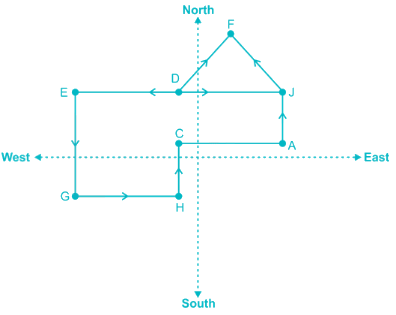Thus it is clearly shown that E is in North-west direction with respect to A.

RRB JE CBT 1 - Full Test 1 - Question 56

Study the following information carefully and answer the given questions.

There are 11 players playing a football match where 2 players got a red card. A is the goalkeeper standing at the eastern point of the ground. A is having the ball and he passes the ball to J who is in the north of A. Then J passes the ball to F who is to his north-west direction. F passes the ball to D who is to his south-west direction. D who is in west direction of J passes the ball to J. Then J kick the ball so that it can reach to player E who is in the west of J. Player D is standing exactly between J and E. E passes the ball to G who is to his south direction. G further passes the ball to H who is in east direction with respect to G. H passes the ball to C who is in the north direction of H such that C is standing to the west of A and south of D. As soon as H passes the ball to C, the referee blows the whistle and it’s half time.

Q. What is the position of E with respect to A?

Detailed Solution for RRB JE CBT 1 - Full Test 1 - Question 56Thus it is clearly shown that D is in North direction with respect to H.

RRB JE CBT 1 - Full Test 1 - Question 57

In the following figure Rectangle represent Cars, Circle represents Sedan, triangle represents SUV and square represent electric. Which of the following represent cars which are electric as well as a sedan.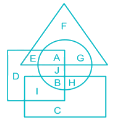Detailed Solution for RRB JE CBT 1 - Full Test 1 - Question 57

1) The rectangle represents Cars.

2) The circle represents Sedan.

3) Triangle represents SUV.

4) Square represents electric.

Cars which are electric, as well as sedan is represented by the letters which are inside the intersection of rectangle, circle and square.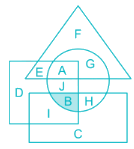Thus, letter ‘B’ represents cars which are electric as well as sedan.

RRB JE CBT 1 - Full Test 1 - Question 58

In the following figure Rectangle represent Hollywood, Circle represent Bollywood, triangle represent Tollywood and Square represents Movies. Which of the following represent Bollywood which are neither Tollywood nor Movies.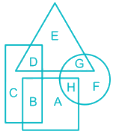Detailed Solution for RRB JE CBT 1 - Full Test 1 - Question 58

1) Rectangle represents Hollywood

2) Circle represent Bollywood

3) Triangle represent Tollywood

4) Square represent Movies.

Bollywood which are neither Tollywood nor Movies is represented by the letters which are inside circle but not in triangle or square.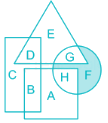Thus letter ‘F’ represents Bollywood which are neither Tollywood nor Movies.

RRB JE CBT 1 - Full Test 1 - Question 59

In the following question, select the number which can be placed at the sign of question mark (?) from the given alternative.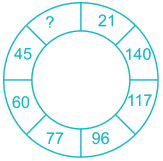Detailed Solution for RRB JE CBT 1 - Full Test 1 - Question 59

The logic here is as follows,

7 × 3 = 21;

8 × 4 = 32;

9 × 5 = 45;

10 × 6 = 60;

11 × 7 = 77;

12 × 8 = 96;

13 × 9 = 117;

14 × 10 = 140

Thus the missing number is 32.

RRB JE CBT 1 - Full Test 1 - Question 60

In a certain code language, '×' represents '+', '÷' represents '×', '-' represents '÷' and '+' represents '-'. Find out the answer to the following question.

42 + 36 – 6 ÷ 15 ×​ 8 ÷ 20 + 20 – 5 = ?

Detailed Solution for RRB JE CBT 1 - Full Test 1 - Question 60

Changing operators as per the given format: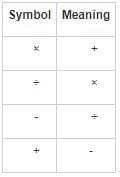Given expression: 42 + 36 – 6 ÷ 15 ×​ 8 ÷ 20 + 20 – 5 = ?

After changing the symbols: 42 – 36 ÷ 6 × 15 + 8 × 20 – 20 ÷ 5

= 42 – 6 × 15 + 160 – 4

= 42 – 90 + 160 – 4

= 108

Hence, “108” is the correct answer.

RRB JE CBT 1 - Full Test 1 - Question 61

Count the number of circles in the following figure.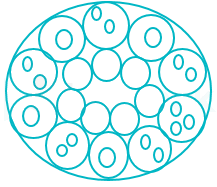Detailed Solution for RRB JE CBT 1 - Full Test 1 - Question 61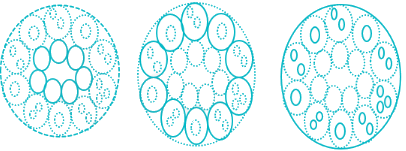There are 7 darken circle in the innermost part of the diagram in the 1st diagram and there are 10 circle darken in the 2nd diagram. And in the 3rd diagram, there are 17 small circles and 1 big outer circle.

Total number of circle = 7 + 10 + 17 + 1 = 35

Hence, there are 35 circles in the given diagram.

RRB JE CBT 1 - Full Test 1 - Question 62

Find out the alternative figure which contains figure (X) as its part.Detailed Solution for RRB JE CBT 1 - Full Test 1 - Question 62

If we look closely, figure(X) is hidden in option figure 1 as shown below.Hence, the answer should be figure 1.

RRB JE CBT 1 - Full Test 1 - Question 63

Directions: The question consists of an assertion and two reasons numbered R (I) and R (II). The student must first determine whether each statement is true and then decide whether the given reasons are correct for the given assertion.

Assertion: Soft skills coaching centres are mushrooming all over India.

Reason (I): People are opting for soft skills training largely.
Reason (II): Other than soft skills, no other skills are useful these days.

Detailed Solution for RRB JE CBT 1 - Full Test 1 - Question 63

Assertion: Soft skills coaching centres are mushrooming all over India → True.

Reason (I): People are opting for soft skills training largely → True and is correct reason for the given assertion.

Reason (II): Other than soft skills, no other skills are useful these days → This statement itself is false. Soft skill is an important part of people’s life but these skills are not the only skills required.

Hence, only R (I) and not R (II) is the correct reason for the given assertion.

RRB JE CBT 1 - Full Test 1 - Question 64

Find the angle between the hour hand and minute hand when the clock shows 3:24 PM.

Detailed Solution for RRB JE CBT 1 - Full Test 1 - Question 64

Using the formula to find the angle between the hour hand and minute hand

θ = 30 H – 11/2 M

Where H = hour hand

And M = minute hand

The hour hand is in between 3 and 4, 30 (3) = 90.

For minute hand

11/2 (24) = 132

The difference between our hand and minute hand will be 132 – 90 = 42 degree

Hence, 42 degree is the correct answer.

RRB JE CBT 1 - Full Test 1 - Question 65

Directions: In the question below a statement is given followed by two conclusions numbered I and II. You have to assume everything in the statement to be true, and then consider the two conclusions together and decide which of them logically follows beyond a reasonable doubt from the information given in the statement.

Statement: Amit got grace marks in Science.

Conclusion I: Amit did not attempt all the questions in Science.
Conclusion II: Some questions were not from the prescribed syllabus.

Detailed Solution for RRB JE CBT 1 - Full Test 1 - Question 65

The fact that Amit got grace marks in science doesn’t mean that he has not attempted all the questions in science. It’s possible that he attempted them but his answers were wrong. Also, the given statement doesn’t reflect anything about the syllabus.

Hence, none of the given conclusions follows.

RRB JE CBT 1 - Full Test 1 - Question 66

The question below consists of a question and two statements numbered I and II given below it. You have to decide whether the data provided in the statements are sufficient to answer the question. Read both the statements and give answer.

Who among the five friends V, W, X, Y and Z is the tallest one?
I) The number of friends taller than V is equal to the number of friends shorter than V.
II) W is not the tallest one but is taller than V and X is smaller than V.

Detailed Solution for RRB JE CBT 1 - Full Test 1 - Question 66

Statement I) Number of friends taller than V is equal to the number of friends shorter than V.

_ > _ > V > _ > _

Statement I alone is not sufficient to answer the question.

Statement II) W is not the tallest one, but is taller than V and X is smaller than V.

_ > W > V > X > _  OR

_ > W > _ > V > X  OR

_ > _ > W > V > X

Statement II alone is also not sufficient to answer the question.

Combining statement I and II,

_ > W > V > X > _

Thus data even in both statement I and II together are not sufficient to answer the question.

RRB JE CBT 1 - Full Test 1 - Question 67

The question below consists of a question and two statements numbered I and II given below it. You have to decide whether the data provided in the statements are sufficient to answer the question. Read both the statements and give answer.

Five persons F, G, H, I and J sitting in a straight line facing north. Who is sitting to the immediate right of H?

I) None of them sits to the left of F and to the right of J.
II) F sits to the immediate left of G, who is not a neighbor of H.

Detailed Solution for RRB JE CBT 1 - Full Test 1 - Question 67

I) None of them sits to the left of F and to the right of J.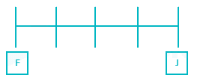Statement I alone is not sufficient to answer the question.

II) F sits to the immediate left of G, who is not a neighbor of H.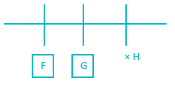Statement II alone is not sufficient to answer the question.

Combining statement I and II,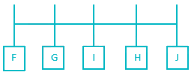On combining statement I and II we can say that J sits to the immediate right of H.

Thus, both the statement I and II are necessary to answer the question.

RRB JE CBT 1 - Full Test 1 - Question 68

There are 5 people B, F, G, C and Q visit the different places namely Shirdi, Goa, Wai, Leh, and Agra in different month June, January, October, September and April of the same year. C travel in the month having 31 days and visits Shirdi. F visited Wai just after the month in which C visited. The person who visited Goa travelled in the month of September. Q travelled in the month having 31 days in it. Number of people travelled before B is equal to the number of people travelled after B. C is not the last one to visit any place in a calendar year.

Q. Which place does Q visit?

Detailed Solution for RRB JE CBT 1 - Full Test 1 - Question 68

5 people: B, F, G, C and Q

Places: Shirdi, Goa, Wai, Leh, and Agra

Month: January, April, June, September and October of the same year.

1) C travel in the month having 31 days and visits Shirdi.

2) C is not the last one to visit any place in a calendar year.

3) Number of people travelled before B is equal to the number of people travelled after B.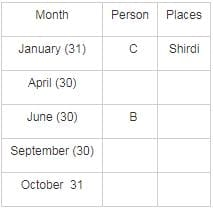4) Q travelled in the month having 31 days in it.

5) F visited Wai just after the month in which C visited.

6) The person who visited Goa travelled in the month of September.

Thus, G is the only person left and he must be travelling in the month of September.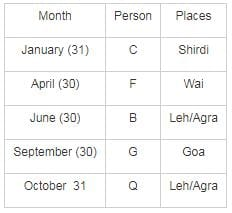Thus Q visits either Leh or Agra.

RRB JE CBT 1 - Full Test 1 - Question 69

Pointing towards non-strikers end, Pujara said, “He is the brother of the daughter of the wife of my brother.” How is the non-striker related to Pujara?

Detailed Solution for RRB JE CBT 1 - Full Test 1 - Question 69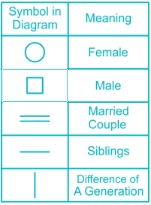According to the question,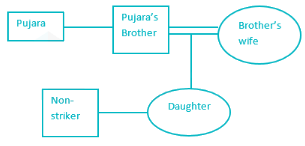Thus Non-striker is the nephew of Pujara.

RRB JE CBT 1 - Full Test 1 - Question 70

Which one of the given responses would be a meaningful order of blood flow from the following?

I. Body
II. Right atrium
III. Left atrium
IV. Left ventricle
V. Lungs
VI. Right ventricle

Detailed Solution for RRB JE CBT 1 - Full Test 1 - Question 70

Given below are the stages of the process of blood flow in a human body.

II. Right atrium → VI. Right ventricle → V. Lungs → III. Left atrium → IV. Left ventricle → I. Body

Thus the correct sequence is II, VI, V, III, IV, I.

RRB JE CBT 1 - Full Test 1 - Question 71

Determine the value of ‘x’ in the equation: x =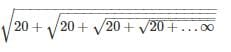Detailed Solution for RRB JE CBT 1 - Full Test 1 - Question 71

Given that, x =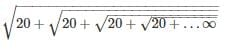⇒ x = √(20 + x)

Squaring both sides,

⇒ x2 = 20 + x

⇒ x2 - x - 20 = 0

⇒ x2 - 5x + 4x - 20 = 0

⇒ x (x - 5) + 4 (x - 5) = 0

x = 5 as x cannot be negative.

Trick

If the given expression is like this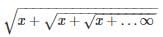Then try to find its factors, and from the two factors which makes x the greater one will be the answer

Here,

x = 20 can be written as = 5 × 4

In this case, 5 is greater than 4 hence the answer will be 5

∴ Value of x = 5

RRB JE CBT 1 - Full Test 1 - Question 72

A Shopkeeper sold big, medium and small-sized chairs for Rs. 650, Rs. 500 and Rs. 400 respectively. The total number of chairs sold were in the ratio 8 ∶ 13 ∶ 4. What is the average cost (in Rs.) of a chair?

Detailed Solution for RRB JE CBT 1 - Full Test 1 - Question 72

Let the total no. of chairs = 8x + 13x + 4x = 25x

Cost of big chairs = 8x × 650 = 5200x

Cost of medium chairs = 13x × 500 = 6500x

Cost of small chairs = 4x × 400 = 1600x

∴ Average cost = (5200x + 6500x + 1600x)/25x = 13300/25 = 532

RRB JE CBT 1 - Full Test 1 - Question 73

The average age of 30 boys in a class is 10 years. If the age of their teacher is also included, the average increases by one year. The teacher's age in years is

Detailed Solution for RRB JE CBT 1 - Full Test 1 - Question 73

Let the total sum of ages of boys in class be x

Then, x/30 = 10 (Given Average)

⇒ x = 300

Now if Teacher’s age is y

⇒ (300 + y)/31 = 11

⇒ 300 + y = 341

⇒ y = 41 years

RRB JE CBT 1 - Full Test 1 - Question 74

The ratio of a number of boys and girls in a school is 2 ∶ 3. If 30% of the boys and 40% of the girls are scholarship holders, then what is the percentage of those students who do not get a scholarship?

Detailed Solution for RRB JE CBT 1 - Full Test 1 - Question 74

Given, ratio of number of boys and girls in a school is 2 : 3.

Let the number of boys and girls be 2a and 3a respectively. Where a is any constant

Given, 30% of the boys and 40% of the girls are scholarship holders.

Total number of boys who are not getting scholarship = 70% of 2a = 1.4a

Total number of girls who are not getting scholarship = 60% of 3a = 1.8a

Total number of students not getting scholarship = 1.4a + 1.8a = 3.2a

% of students not getting the scholarship =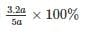∴ % of students not getting scholarship = 64%

RRB JE CBT 1 - Full Test 1 - Question 75

If the price of sugar is raised by 25%, find by how much percent a householder must reduce his consumption of sugar so as not to increase his expenditure?

Detailed Solution for RRB JE CBT 1 - Full Test 1 - Question 75

Let the initial consumption of sugar be Y units and the initial price of sugar per kg be Rs. X. Thus,

The initial expenditure of household = X × Y

Given: the price of sugar increased by 25%. Thus,

⇒New price of sugar per kg =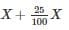= 1.25X

Let the new consumption to keep the expenditure same be Y’ units. Hence,

New expenditure = 1.25X × Y’

Since the expenditures are same,

∴ XY = 1.25XY’

⇒ Y = 1.25Y’

Also, Reduction in consumption = Y – Y’

= 1.25Y’ – Y’

= 0.25Y’

⇒ Reduction percentage =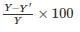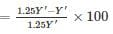= 20%

RRB JE CBT 1 - Full Test 1 - Question 76

Incomes of X and Y are in the ratio 7 ∶ 8. Their expenditures are in the ratio 4 ∶ 5. If both individually save Rs. 960 at the end of the month, then what is the income (in Rs) of X?

Detailed Solution for RRB JE CBT 1 - Full Test 1 - Question 76

Let the incomes of X and Y be 7a and 8a

Let the expenditures of X and Y be 4b and 5b.

Then the income of both will be,

⇒ 7a = 4b + 960     ----(1)

⇒ 8a = 5b + 960     ----(2)

By solving the above equation, we get

a = 320 and b = 320

The income of X = 7a = 7 × 320 = 2240.

RRB JE CBT 1 - Full Test 1 - Question 77

If a ∶ b = 2 ∶ 3, b ∶ c = 4 ∶ 5 and c ∶ d = 6 ∶ 7, then a ∶ d = ?

Detailed Solution for RRB JE CBT 1 - Full Test 1 - Question 77

a : b = 2 : 3

⇒ a/b = 2/3

⇒ b = 3a/2

b : c = 4 : 5

⇒ b/c = 4/5

⇒ 3a/2 : c = 4 : 5

⇒ c = 15a/8

c : d = 6 : 7

⇒c/d = 6/7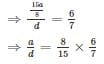⇒ a/d = 16/35

⇒ a : d = 16 : 35

RRB JE CBT 1 - Full Test 1 - Question 78

A jar contains a blend of a fruit juice and water in the ratio 5 ∶ x. When 1 liter of water is added to 4 liters of the blend the ratio of fruit juice to water becomes 1 ∶ 1. What is the value of x?

Detailed Solution for RRB JE CBT 1 - Full Test 1 - Question 78

A jar contains a blend of a fruit juice and water in the ratio 5 : x

Quantity of fruit juice in 4 litres of blend = 4[5/(5 + x)] = 20/(5 + x)

⇒ Quantity of water in 4 litres of blend = 4[x/(5 + x)] = 4x/(5 + x)

According to questions

20/(5 + x) : {4x/(5 + x) + 1} = 1 : 1

⇒ 20 - 4x = 5 + x

∴ x = 3

RRB JE CBT 1 - Full Test 1 - Question 79

Find the unit place digit in the expression given below.
1! + 2! + 3! + 4! + ....... + 20!

Detailed Solution for RRB JE CBT 1 - Full Test 1 - Question 79

As, 5! = 1 × 2 × 3 × 4 × 5 will have 0 as its unit digit because of the presence of (2 × 5). Hence all factorials above it will have 0 as their unit digit

⇒ 1! + 2! + 3! + 4! = 1 + 2 + 6 + 24 = 33

∴ Unit digit of (1! + 2! + 3! + 4! + ....... + 20!) = Unit digit of (1! + 2! + 3! + 4!) = 3

RRB JE CBT 1 - Full Test 1 - Question 80

Find the least number required to be added to 3105 so that it is exactly divisible by 3, 4, 5 and 6.

Detailed Solution for RRB JE CBT 1 - Full Test 1 - Question 80

LCM of 3, 4, 5 and 6 = 2 × 3 × 2 × 5 × 1 × 1 = 60

On dividing 60 with 3105, we get remainder 45

∴ Number to be added = 60 - 45 = 15

Find the least number required to be added to 3105 so that it is exactly divisible by 3, 4, 5 and 6.

RRB JE CBT 1 - Full Test 1 - Question 81

If x3 + y3 = 20 and x + y = 5, then the value of

Detailed Solution for RRB JE CBT 1 - Full Test 1 - Question 81

Given x3 + y3 = 20 and x + y = 5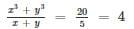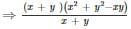= 4 ⇒ x+ y− xy = 4
⇒ (x+y)2 − 3xy = 4 ⇒ 3xy = 52 − 4 = 25 − 4 = 21
xy = 21/3 = 7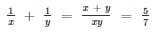RRB JE CBT 1 - Full Test 1 - Question 82

If x = √5 + 2, then the value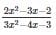is equal to

Detailed Solution for RRB JE CBT 1 - Full Test 1 - Question 82

x = √5 + 2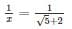On rationalizing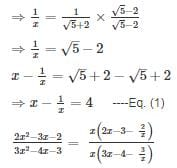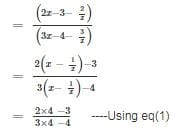= 5/8

= .625

RRB JE CBT 1 - Full Test 1 - Question 83

If x4 + y4 = x2y2 , Find x6 + y6

Detailed Solution for RRB JE CBT 1 - Full Test 1 - Question 83

x6 + y6 = (x2)3 + (y2)3
x6+y6=(x2+y2)(x4+y4−x2y2)

x6+y6=(x2+y2)(x2y2−x2y2)x6+y6=(x2+y2)(x2y2−x2y2)

x6 + y6 = 0

RRB JE CBT 1 - Full Test 1 - Question 84

A person sells 36 oranges per rupee and suffers a loss of 4%. How many oranges per rupee has to be sold to have a gain of 8%?

Detailed Solution for RRB JE CBT 1 - Full Test 1 - Question 84

SP of 1 orange is 1/36 and it suffers 4% loss.

Let CP be x.

⇒ (96/100)x = 1/36

⇒ x = 100/(36 × 96)

To make profit of 8%

He should sell = 108/(36 × 96) = 1/32

∴ 32 oranges per rupee

RRB JE CBT 1 - Full Test 1 - Question 85

Jay and Jatin invested Rs. 80000 and Rs. 60000 respectively in a business. After 4 months of investing, Jatin invested Rs. 10000 more, while Jay withdrew Rs. 10000 from his investment. At the end of the year, what is the ratio of their profits?

Detailed Solution for RRB JE CBT 1 - Full Test 1 - Question 85

Jay’s total investment = 80000 × 4 + 70000 × 8 = 320000 + 560000 = 880000

Jatin’s total investment = 60000 × 4 + 70000 × 8 = 240000 + 560000 = 800000

∵ Ratio of their profits = ratio of their total investment

∴ Ratio of their profits = 880000 ∶ 800000 = 11 ∶ 10

RRB JE CBT 1 - Full Test 1 - Question 86

A certain sum becomes 'k' times in 6 years at compound interest. In 24 years it will become how many times?

Detailed Solution for RRB JE CBT 1 - Full Test 1 - Question 86

We know the formula for compound interest –

CI = [P{1 + r/100}t – 1]

Where,

CI = Compound interest

P = Principal

R = Rate of interest

T = Time period

According to the question,

⇒ kP – P = [P {1 + r/100}6 – 1]

⇒ kP = P {1 + r/100}6          ----- (1)

∴ Total sum becomes after 24 year

⇒ P {1 + r/100}24 = P [{1 + r/100}6]4

Using equation (1)

= Pk4

RRB JE CBT 1 - Full Test 1 - Question 87

A sum on compound interest amounts to Rs. 5618 in 2 years and Rs. 5786.54 in 3 years. Find the sum.

Detailed Solution for RRB JE CBT 1 - Full Test 1 - Question 87

Note : Compound Interest for 1 year is equal to the Simple Interest for 1 year

Interest on Rs. 5618 in 1 year = Rs. (5786.54 - 5618) = Rs. 168.54

Rate =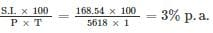Let the sum be Rs. y.

Amount in 2 years =

y(1+3/100)= 5618

By solving, we get

Sum, y = Rs. 5295.50

RRB JE CBT 1 - Full Test 1 - Question 88

One train is travelling 45 km an hour and other is at 10 meters a second. The Ratio of the speed of the two trains is.

Detailed Solution for RRB JE CBT 1 - Full Test 1 - Question 88

⇒ Speed of Train 1 = 45 kmph = 45 × 1000 meters per hour = 45000 meters / hour

= 45000 / 3600 meters per second

= 12.5 meters a second

According to Question the Speed of Train 2 = 10 meters a second

⇒ Ratio = Speed Of Train 1 : Speed of Train 2

⇒ Ratio = 12.5/10 = 5 : 4

RRB JE CBT 1 - Full Test 1 - Question 89

A man can row 10 km/hr in still water. If the speed of the current is 2 km/hr, he takes 3 hours more in upstream than in the downstream. What is the distance (in km)?

Detailed Solution for RRB JE CBT 1 - Full Test 1 - Question 89

Speed of the man in still water = 10 km/h

Speed of the current = 2 km/h

Speed of the man along the current = (10 + 2) km/h = 12 km/h

Speed of the man against the current = (10 – 2) km/h = 8 km/h

Given,

He takes 3 hours more in upstream than in the downstream

Let, The distance is x km = x/8 – x/12 = 3

⇒ x = 72 km

RRB JE CBT 1 - Full Test 1 - Question 90

A 450-meter long train crosses a bridge 650 meters long in 36 seconds. What is speed (in km/hr) of the train?

Detailed Solution for RRB JE CBT 1 - Full Test 1 - Question 90

Length of train = 450 m

Length of bridge = 650 m

To cross a bridge train has to cover a distance which is sum of the length of the train and length of the bridge.

∴ Distance covered to cross the bridge

= 450 m + 650 m

= 1100 m

Time required = 36 seconds

∴ Speed = Distance covered/Required time = 1100/36 = (1100/1000)/(36/3600)

= 110 km/hr

RRB JE CBT 1 - Full Test 1 - Question 91

Tap A can fill a tank in 9 hours and tap B can fill the same tank in 6 hours. Tap C can empty the same full tank in 4 hours. If all the taps are opened together, then what portion of the tank will be filled after 6 hours?

Detailed Solution for RRB JE CBT 1 - Full Test 1 - Question 91

A can fill the tank in = 9 hours

∴ In 1 hour A can fill = 1/9

B can fill the tank in = 6 hours

∴ In 1 hour B can fill = 1/6

C can empty the tank in = 4 hours

∴ In 1 hour C can empty = 1/4

∴ In 6 hours they together can fill ,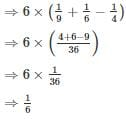RRB JE CBT 1 - Full Test 1 - Question 92

A garrison of 160 men has provision for 40 days. At the end of 10 days, 40 more men joined them. How many days could the soldiers sustain themselves if they had joined on the first day itself?

Detailed Solution for RRB JE CBT 1 - Full Test 1 - Question 92

160 men can last for 40 days

1 man can last for = 40 × 160 days

Total number of men = 160 + 40 = 200

200 soldiers can last for = (30 × 160/200) = 24 days

RRB JE CBT 1 - Full Test 1 - Question 93

If the area of a triangle with base 15 cm is equal to the area of a square with side 15 cm. The altitude of the triangle will be

Detailed Solution for RRB JE CBT 1 - Full Test 1 - Question 93

Let the altitude of the triangle be ‘h’ centimeters.

We know that, Area of a triangle = ½ × base × height

And, area of a square = side2

According to question, Area of the given triangle = Area of the given square

⇒1/2 × 15 × h = 152

⇒ h = 30 cm

Hence, the height of the triangle is 30 centimeters.

RRB JE CBT 1 - Full Test 1 - Question 94

The ratio of the volumes of a right circular cylinder and a sphere is 3 ∶ 2. If the radius of the sphere is double the radius of the base of the cylinder; find the ratio of the curved surface area of the cylinder and surface area of the sphere?

Detailed Solution for RRB JE CBT 1 - Full Test 1 - Question 94

Let radius of cylinder be r and radius of sphere be R

Volume of a right circular cylinder = πr2h

Volume of a sphere = (4/3)πR3

Given,

R = 2r

Ratio of the volumes of a right circular cylinder and a sphere is 3 : 2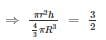⇒ h = 16r

Curved Surface area of a right circular cylinder = 2πrh

Surface area of the sphere = 4πR2

∴ Ratio of the surface areas of the cylinder and the sphere =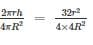= 2 : 1

RRB JE CBT 1 - Full Test 1 - Question 95

The side BC of ΔABC is produced to D. If ∠ACD = 114° and ∠ABC = (1/2)∠BAC, then what is the value (in degrees) of ∠BAC?

Detailed Solution for RRB JE CBT 1 - Full Test 1 - Question 95

Let ∠ABC be x then ∠BAC = 2x

Using Exterior angle property

⇒ ∠CAB + ∠ABC = ∠ ACD

⇒ 2x + x = 114

⇒ x = 38

⇒ ∠BAC = 2x = 2 × 38 = 76

∴ ∠BAC is 76

RRB JE CBT 1 - Full Test 1 - Question 96

In the given figure, PQR is a triangle in which, PQ = 24 cm, PR = 12 cm and altitude PS = 8 cm. If PT is the diameter of the circumcircle, then what is the length (in cm) of circum-radius?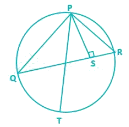Detailed Solution for RRB JE CBT 1 - Full Test 1 - Question 96

If a, b, and c are the three sides of a triangle, then the circum-radius ‘R’ is given as,

R = abc/4A

Where A = area of triangle = (base × height)/2

Given, height = 8 cm

Let base = QR = x cm

⇒ Area of triangle = A = 8x/2 = 4x

The other two sides of triangle are of lengths 24 cm and 12 cm,

∴ Circum-radius of triangle = (24 × 12 × x)/(4 × 4x) = 18 cm

RRB JE CBT 1 - Full Test 1 - Question 97

If x = cos2θ + sec2θ, then which statement is correct -

Detailed Solution for RRB JE CBT 1 - Full Test 1 - Question 97

x = cos2θ + sec2 θ

For a cos2θ + b sec2θ

Min value =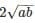So x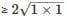∴ x ≥ 2

Alternate Solution:

Arithmetic mean ≥ Geometric mean

(cos2θ + sec2 θ)/2 ≥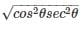cos2θ + sec2 θ ≥ 2√1

∴ x ≥ 2

RRB JE CBT 1 - Full Test 1 - Question 98

If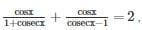then the value of x -

Detailed Solution for RRB JE CBT 1 - Full Test 1 - Question 98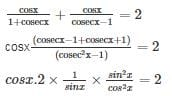∴ tanx =1

∴ x = π/4

RRB JE CBT 1 - Full Test 1 - Question 99

If the sum of the interior angles of a regular polygon is 540o then how many sides does it have?

Detailed Solution for RRB JE CBT 1 - Full Test 1 - Question 99

Let the regular polygon has n sides. We also know that the sum of all the interior angles of a regualr polygon with n sides is (n - 2) × 180°

According to the question,

⇒ (n - 2) × 180° = 540°

⇒ n - 2 = 3

∴ n = 5

RRB JE CBT 1 - Full Test 1 - Question 100

Directions: This is a pie chart for the data on A, B, O, AB blood groups of 150 donors. Observe the pie chart and answer the questions.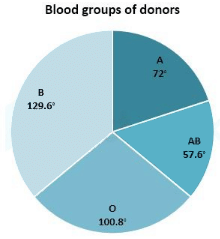Q. What percentage of the donor is having blood group 'AB'?

Detailed Solution for RRB JE CBT 1 - Full Test 1 - Question 100

Required percentage = 57.6/360 × 100 = 16%

## RRB JE (Railways Junior Engineer) CBT Mock Test Series

19 tests
Information about RRB JE CBT 1 - Full Test 1 Page
In this test you can find the Exam questions for RRB JE CBT 1 - Full Test 1 solved & explained in the simplest way possible. Besides giving Questions and answers for RRB JE CBT 1 - Full Test 1, EduRev gives you an ample number of Online tests for practice

## RRB JE (Railways Junior Engineer) CBT Mock Test Series

19 tests(Scan QR code)